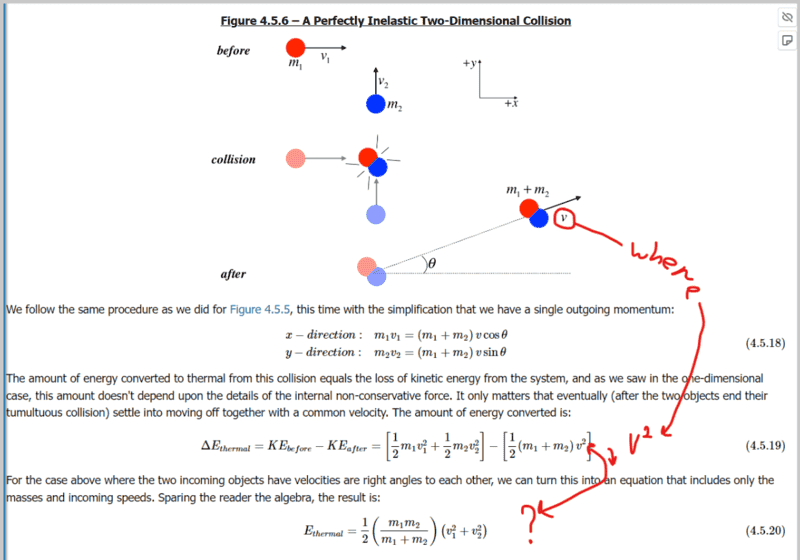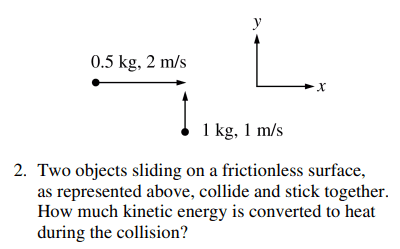# Perpendicular inelastic collision problem

• NODARman

#### NODARman

Homework Statement
Where did "v" go?
Relevant Equations
.
I still don't get it where did "v" go.
I'm trying to solve the problem that is on the second image.Second image.•Delta2
Yes well your book doesn't show all the in between steps on how exactly it derives that result. It just states "Sparing the reader the algebra".

What is done in the in between steps (which you should try to work out by yourself, I ll just outline the steps) is that the system of equations 4.5.18 (two equations with two unknowns, the common velocity and the angle) is solved and then once you solve it and find ##v## (##v## will be a function of ##m_1,m_2, v_1,v_2##) then ##v## it is replaced in equation 4.5.19 and then after some algebra you end up with equation 4.5.20.

•NODARman
Homework Statement:: Where did "v" go?
Relevant Equations:: .

I still don't get it where did "v" go.
I'm trying to solve the problem that is on the second image.
View attachment 304812

Second image.
View attachment 304813
There's a general result (which can be derived) for energy loss in a 1 dimensional inelastic (collide and coalesce) collision: $$\Delta E= \frac{1}{2} \mu \Delta v^2$$ where ##\mu## is the reduced mass of the colliding objects and ##\Delta v## their relative velocity: $$\mu = \frac{m_1m_2}{m_1+m_2}$$It looks like there's nothing different here except that ##\Delta v## is replaced by the vector difference of the two velocities.

•NODARman